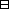## Group Theory and Linear Algebra

Last updated: 24 September 2014

## Lecture 22: Cosets and quotient groups

Let $G$ be a group. A subgroup of $G$ is a subset $H\subseteq G$ such that

 (a) If ${h}_{1},{h}_{2}\in H$ then ${h}_{1},{h}_{2}\in H,$ (b) $1\in H,$ (c) If $h\in H$ then ${h}^{-1}\in H\text{.}$

Let $H$ be a subgroup of $G\text{.}$ A coset of $H$ in $G$ is a subset $gH={gh | h∈H}$ with $g\in G\text{.}$

$G = { , , , , , } =S3, H = { , } .$ Then $·H = { , , } , ·H = { , } , ·H = { , } , ·H = { , } , ·H = { , } , ·H = { , } .$ There are really only $3$ cosets here since $·H= ·H= { , } , ·H= ·H= { , } , ·H= ·H= { , } .$ $G}{H}=\left\{gH | g\in G\right\}$ is the set of cosets of $H$ in $G\text{.}$ In our example $S3H = { ·H, ·H, ·H } = { { , } , { , } , { , } } .$

Let $G$ be a group and let $H$ be a subgroup of $G\text{.}$ Let $g\in G\text{.}$

 (a) $\text{Card}\left(gH\right)=\text{Card}\left(H\right)\text{.}$ (b) $G}{H}$ is a partition of $G\text{.}$

A partition of a set $S$ is a collection $𝒮$ of subsets of $S$ such that

 (a) The union of the sets in $𝒮$ is $S,$ (b) If ${U}_{1},{U}_{2}\in 𝒮$ then ${U}_{1}={U}_{2}$ or ${U}_{1}\cap {U}_{2}=\varnothing \text{.}$

Let $G$ be a group and let $H$ be a subgroup of $G\text{.}$ Then $Card(G)= Card(GH) Card(H).$Proof of the proposition.

(a) To show: $\text{Card}\left(gH\right)=\text{Card}\left(H\right)\text{.}$
To show: There exists a bijective function $f:H\to gH\text{.}$
Let $f: H ⟶ gH h ⟼ gh .$ To show: $f$ is bijective.
To show: There exists a function $\phi :gH\to H$ such that $\phi \circ f={\text{id}}_{H}$ and $f\circ \phi ={\text{id}}_{gH}\text{.}$
Let $φ: gH ⟶ H x ⟼ g-1x$ so that $\phi \left(x\right)={g}^{-1}x\text{.}$
To show:
 (aa) $\phi \circ f={\text{id}}_{H}\text{.}$ (ab) $f\circ \phi ={\text{id}}_{gH}\text{.}$
 (aa) To show: If $h\in H$ then $\left(\phi \circ f\right)\left(h\right)={\text{id}}_{H}\left(h\right)\text{.}$ Assume $h\in H\text{.}$ To show: $\left(\phi \circ f\right)\left(h\right)={\text{id}}_{H}\left(h\right)\text{.}$ $(φ∘f)(h)= φ(f(h))= φ(gh)= g-1gh=h= idH(h).$ (ab) To show: If $x\in gH$ then $\left(f\circ \phi \right)\left(x\right)={\text{id}}_{gH}\left(x\right)\text{.}$ Assume $x\in gH\text{.}$ To show: $\left(f\circ \phi \right)\left(x\right)={\text{id}}_{gH}\left(x\right)\text{.}$ $(f∘φ)(x)= f(φ(x))= f(g-1x)= gg-1x=x= idgH(x).$
(b)
To show: $G}{H}$ is a partition of $G\text{.}$
To show:
 (ba) $\bigcup _{g\in G}gH=G\text{.}$ (bb) If ${g}_{1},{g}_{2}\in G$ then ${g}_{1}H={g}_{2}H$ or ${g}_{1}H\cap {g}_{2}H=\varnothing \text{.}$
(ba) To show: If $x\in G$ then there exists $g\in G$ such that $x\in gH\text{.}$
Assume $x\in G\text{.}$
To show: There exists $g\in G$ such that $x\in gH\text{.}$
Let $g=x\text{.}$
To show: $x\in gH\text{.}$ $x=·1∈xH,$ since $1\in H\text{.}$
(bb) Assume ${g}_{1},{g}_{2}\in G$ and ${g}_{1}H\cap {g}_{2}H\ne \varnothing \text{.}$
To show: ${g}_{1}H={g}_{2}H\text{.}$
Since ${g}_{1}H\cap {g}_{2}H\ne \varnothing$ there exists $x\in {g}_{1}H\cap {g}_{2}H\text{.}$
Let ${h}_{1},{h}_{2}\in H$ be such that $x={g}_{1}{h}_{1}$ and $x={g}_{2}{h}_{2}\text{.}$
To show: ${g}_{1}H={g}_{2}H\text{.}$
To show:
 (bba) ${g}_{1}H\subseteq {g}_{2}H\text{.}$ (bbb) ${g}_{2}H\subseteq {g}_{1}H\text{.}$
 (bba) To show: If $y\in {g}_{1}H$ then $y\in {g}_{2}H\text{.}$ Assume $y\in {g}_{1}H\text{.}$ Then there exists $h\in H$ such that $y={g}_{1}H\text{.}$ To show: $y\in {g}_{2}H\text{.}$ $y=g1h=g1h1h1-1 h=xh1-1h=g2h2 h1-1h∈g2H,$ since $H$ is a subgroup. (bbb) To show: If $z\in {g}_{2}H$ then $z\in {g}_{1}H\text{.}$ Assume $z\in {g}_{2}H\text{.}$ Then there exists $h\prime \in H$ such that $z={g}_{2}h\prime \text{.}$ To show: $z\in {g}_{1}H\text{.}$ $z=g2h′=g2h2 h2-1h′=x h2-1h′=g1h1 h2-1h′∈g1H,$ since $H$ is a subgroup.
So ${g}_{1}H={g}_{2}H\text{.}$
So $G}{H}$ is a partition of $G\text{.}$

$\square$

## Notes and References

These are a typed copy of Lecture 22 from a series of handwritten lecture notes for the class Group Theory and Linear Algebra given on September 13, 2011.